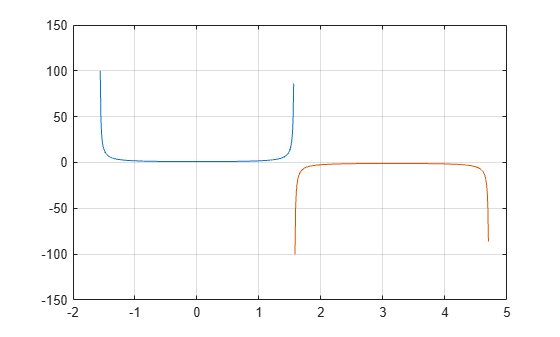# sec

## Syntax

``Y = sec(X)``

## Description

example

````Y = sec(X)` returns the secant of the elements of `X`. The `sec` function operates element-wise on arrays. The function accepts both real and complex inputs. For real values of `X`, `sec(X)` returns real values in the interval [-∞, -1] and [1, ∞].For complex values of `X`, `sec(X)` returns complex values. ```

## Examples

collapse all

Plot the secant over the domain $-\pi /2 and $\pi /2 .

```x1 = -pi/2+0.01:0.01:pi/2-0.01; x2 = pi/2+0.01:0.01:(3*pi/2)-0.01; plot(x1,sec(x1),x2,sec(x2)), grid on```Calculate the secant of the complex angles in vector `x`.

```x = [-i pi+i*pi/2 -1+i*4]; y = sec(x)```
```y = 1×3 complex 0.6481 + 0.0000i -0.3985 + 0.0000i 0.0198 - 0.0308i ```

## Input Arguments

collapse all

Input angle in radians, specified as a scalar, vector, matrix, or multidimensional array.

Data Types: `single` | `double`
Complex Number Support: Yes

## Output Arguments

collapse all

Secant of input angle, returned as real-valued or complex-valued scalar, vector, matrix or multidimensional array.

collapse all

### Secant Function

The secant of an angle, α, defined with reference to a right angled triangle isThe secant of a complex argument, α, is

`$\text{sec}\left(\alpha \right)=\frac{2}{{e}^{i\alpha }+{e}^{-i\alpha }}\text{\hspace{0.17em}}.$`

## Tips

• In floating-point arithmetic, `sec` is a bounded function. That is, `sec` does not return values of `Inf` or `-Inf` at points of divergence that are multiples of `pi`, but a large magnitude number instead. This stems from the inaccuracy of the floating-point representation of π.